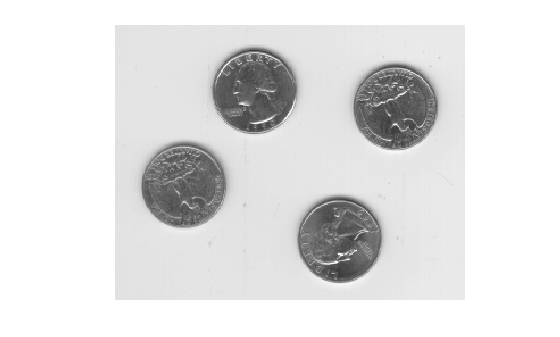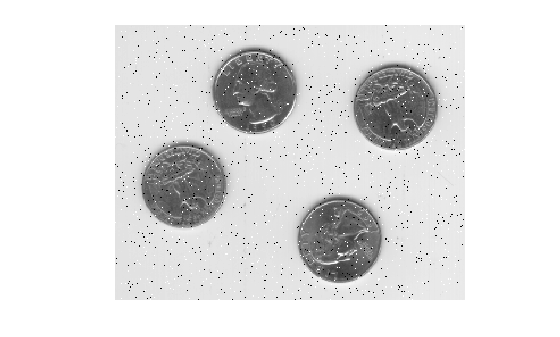imnoise

Description

J = imnoise(I,'gaussian') adds zero-mean, Gaussian white noise with variance of 0.01 to grayscale image I.

J = imnoise(I,'gaussian',m) adds Gaussian white noise with mean m and variance of 0.01.

J = imnoise(I,'gaussian',m,var_gauss) adds Gaussian white noise with mean m and variance var_gauss.

J = imnoise(I,'localvar',var_local) adds zero-mean, Gaussian white noise of local variance var_local.

J = imnoise(I,'localvar',intensity_map,var_local) adds zero-mean, Gaussian white noise. The local variance of the noise, var_local, is a function of the image intensity values in I. The mapping of image intensity value to noise variance is specified by the vector intensity_map.

J = imnoise(I,'salt & pepper') adds salt and pepper noise, with default noise density 0.05. This affects approximately 5% of pixels.

example

J = imnoise(I,'salt & pepper',d) adds salt and pepper noise, where d is the noise density. This affects approximately d*numel(I) pixels.

J = imnoise(I,'speckle') adds multiplicative noise using the equation J = I+n*I, where n is uniformly distributed random noise with mean 0 and variance 0.05.

J = imnoise(I,'speckle',var_speckle) adds multiplicative noise with variance var_speckle.

Examples

collapse all

Read a grayscale image and display it.

imshow(I)Add salt and pepper noise, with a noise density of 0.02, to the image. Display the result.

J = imnoise(I,'salt & pepper',0.02);
imshow(J)Input Arguments

collapse all

Grayscale image, specified as a numeric array of any dimensionality.

imnoise expects pixel values of data type double and single to be in the range [0, 1]. You can use the rescale function to adjust pixel values to the expected range. If your image is type double or single with values outside the range [0,1], then imnoise clips input pixel values to the range [0, 1] before adding noise.

Note

For Poisson noise, imnoise does not support images of data type int16.

Data Types: single | double | int16 | uint8 | uint16

Mean of Gaussian noise, specified as a numeric scalar.

Variance of Gaussian noise, specified as a numeric scalar.

Local variance of Gaussian noise, specified as one of the following:

• A numeric matrix of the same size as I.

• A numeric vector the same length of intensity_map.

Intensity values that are mapped to Gaussian noise variance, specified as a numeric vector. The values are normalized to the range [0, 1].

You can plot the functional relationship between noise variance var_local and image intensity using the command plot(intensity_map,var_local).

Noise density for salt and pepper noise, specified as a numeric scalar. The noise is applied to approximately d*numel(I) pixels.

Variance of multiplicative noise, specified as a numeric scalar.

Output Arguments

collapse all

Noisy image, returned as a numeric matrix of the same data type as input image I. For images of data type double or single, the imnoise function clips output pixel values to the range [0, 1] after adding noise.

Algorithms

• The mean and variance parameters for 'gaussian', 'localvar', and 'speckle' noise types are always specified as if the image were of class double in the range [0, 1]. If the input image is a different class, the imnoise function converts the image to double, adds noise according to the specified type and parameters, clips pixel values to the range [0, 1], and then converts the noisy image back to the same class as the input.

• The Poisson distribution depends on the data type of input image I:

• If I is double precision, then input pixel values are interpreted as means of Poisson distributions scaled up by 1e12. For example, if an input pixel has the value 5.5e-12, then the corresponding output pixel will be generated from a Poisson distribution with mean of 5.5 and then scaled down by 1e12.

• If I is single precision, the scale factor used is 1e6.

• If I is uint8 or uint16, then input pixel values are used directly without scaling. For example, if a pixel in a uint8 input has the value 10, then the corresponding output pixel will be generated from a Poisson distribution with mean 10.

• To add 'salt & pepper' noise with density d to an image, imnoise first assigns each pixel a random probability value from a standard uniform distribution on the open interval (0, 1).

• For pixels with probability value in the range (0, d/2), the pixel value is set to 0. The number of pixels that are set to 0 is approximately d*numel(I)/2.

• For pixels with probability value in the range [d/2, d), the pixel value is set to the maximum value of the image data type. The number of pixels that are set to the maximum value is approximately d*numel(I)/2.

• For pixels with probability value in the range [d, 1), the pixel value is unchanged.